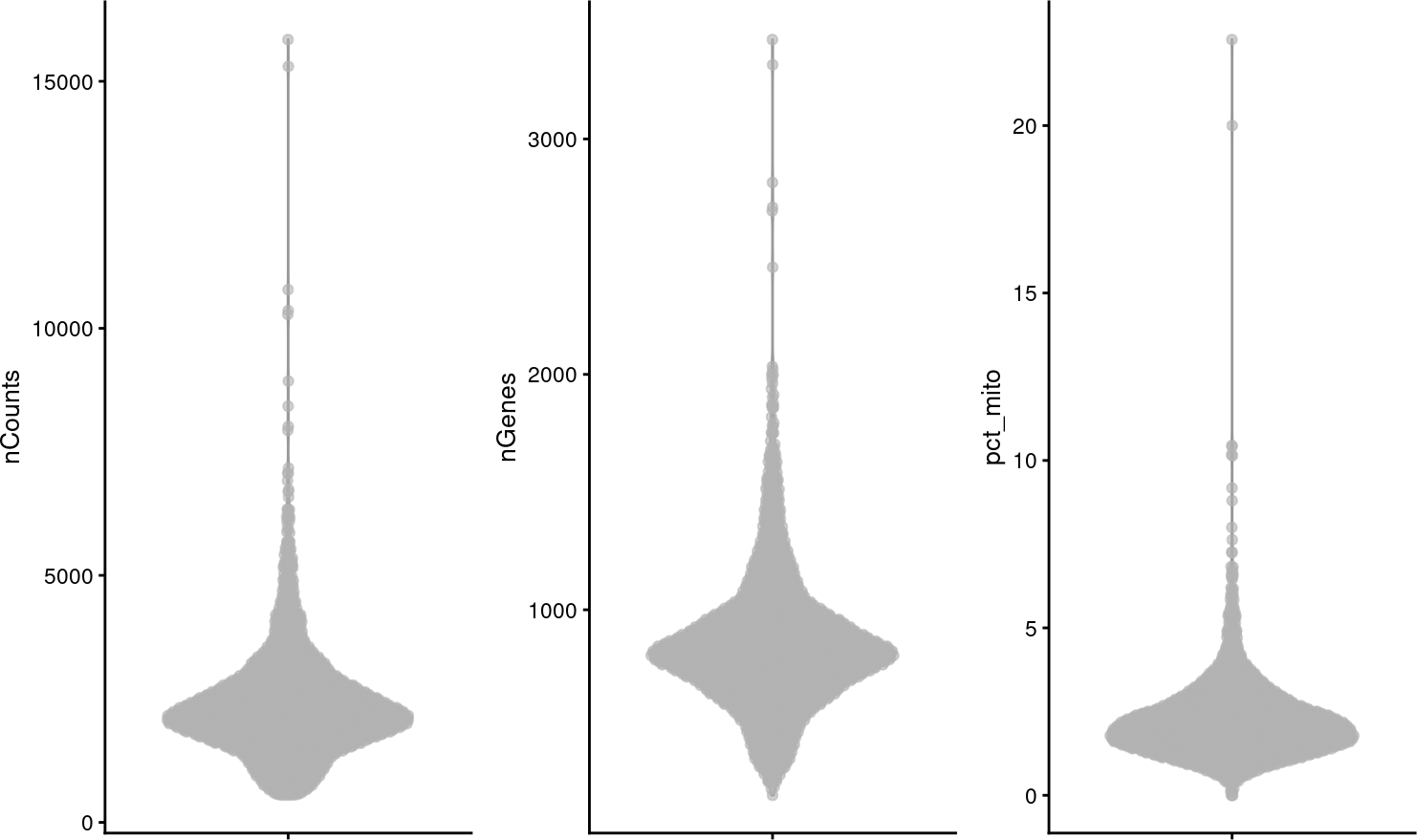# 1 Introduction

UMAP is commonly used in scRNA-seq data analysis as a visualization tool projecting high dimensional data onto 2 dimensions to visualize cell clustering. However, UMAP is prone to showing spurious clustering and distorting distances (Chari, Banerjee, and Pachter 2021). Moreover, UMAP shows clustering that seems to correspond to graph-based clusters from Louvain and Leiden because the k nearest neighbor graph is used in both clustering and UMAP. We have developed `concordex` as a quantitative alternative to UMAP cluster visualization without the misleading problems of UMAP. This package is the R implementation of the original Python command line tool.

In a nutshell, `concordex` finds the proportion of cells among the k nearest neighbors of each cell with the same cluster or label as the cell itself. This is computed across all labels and the average of all labels is returned as a metric that indicates the quality of clustering. To see if this is significant, the labels are permuted to estimate a null distribution and the actual observed value is compared to the simulated values. If the clustering separates cells well, then the observed value should be much higher than the simulated values, i.e. the neighborhood of each cell is more dominated by cells of the same label as the cell of interest than by chance.

``````library(concordexR)
library(TENxPBMCData)
library(BiocNeighbors)
library(bluster)
library(scater)
library(patchwork)
library(ggplot2)
theme_set(theme_bw())``````

# 2 Preprocessing

In this vignette, we demonstrate the usage of `concordex` on a human peripheral blood mononuclear cells (PBMC) scRNA-seq dataset from 10X Genomics. The data is loaded as a `SingleCellExperiment` object.

``````sce <- TENxPBMCData("pbmc3k")
#> see ?TENxPBMCData and browseVignettes('TENxPBMCData') for documentation

Here we plot the standard QC metrics: total number of UMIs detected per cell (`nCounts`), number of genes detected (`nGenes`), and percentage of UMIs from mitochondrially encoded genes (`pct_mito`).

``````sce\$nCounts <- colSums(counts(sce))
sce\$nGenes <- colSums(counts(sce) > 0)
mito_inds <- grepl("^MT-", rowData(sce)\$Symbol_TENx)
sce\$pct_mito <- colSums(counts(sce)[mito_inds,])/sce\$nCounts * 100``````
``````plotColData(sce, "nCounts") +
plotColData(sce, "nGenes") +
plotColData(sce, "pct_mito")````````````p1 <- plotColData(sce, x = "nCounts", y = "nGenes") +
geom_density2d()
p2 <- plotColData(sce, x = "nCounts", y = "pct_mito") +
geom_density2d()
p1 + p2``````